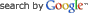DEPARTMENT OF ELECTRONIC & ELECTRICAL ENGINEERING LIQUID CRYSTAL MODELLINGINFORMATION FOR

INFORMATION

PROJECTS# Liquid Crystal Hydrodynamics with Variable OrderWhen disclinations are present, the LC ordering becomes biaxial near the core and the order parameter drops (as represented by the background colour of the figure to the right). We have developed Finite Element discretisations of the Qian-Sheng Equations in both 2D and 3D. The Qian-Sheng equations are a generalisation of Ericksen-Leslie theory for LC hydrodynamics to include changes in the order parameter . The Finite Element Method is well suited to resolve the rapid variations in order parameter about disclination whilst still being able to model large container sizes.

• Three elastic constants
• Flexo-electric effect
• Anisotropic weak anchoring 

## Theory

The order and the orientation of the liquid crystal are represented by a symmetric and traceless tensor, the Q-tensor: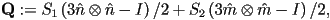where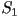and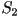represent the degree of order about the vectors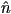and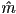respectively.

The equilibrium Q-tensor field minimizes the Landau-de Gennes (LdG) free energy functional: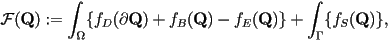where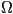is an open bounded subset of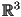with boundary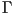. The free-energy densities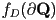and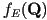are due to elastic and electrostatic contributions respectively and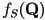is the surface free-energy density. The bulk free-energy density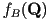constrains the degree of order of the LC: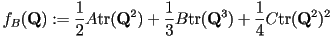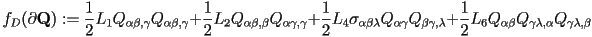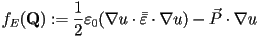where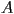,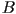and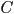are thermotropic constants,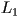to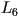are elastic constants,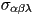is the Levi-Civita anti-symmetric tensor and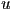is the electric potential. Here, the saddle-splay modulus has been neglected. The flexoelectric polarization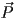can be defined as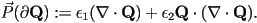The surface contribution takes the form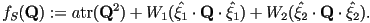This is an anisotropic anchoring energy; a different energy penalty can be assigned to azimuthal and zenithal perturbations from the preferred (easy) alignment direction. The first term is necessary to maintain the surface order parameter,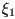and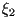are unit vectors orthogonal to the easy direction and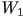and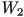are anchoring strengths. A more complete description of the anchoring types supported by this expression and a means to calculate the coefficient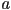can be found in .

## Implementation 

The implementation is well suited to the modelling of defect movement in realistically sized geometries, via the following features:

• Adaptive meshing scheme based on an empirical error estimate
• Crank-Nicholson time integration using a variable time step
• Matrices calculated using Gauss integration, allowing curved edge elements to be used

This implicit scheme leads to a larger memory use than the constant order program. However, the rapid spatial variations in the order parameter near disclinations severely limits the time step for explicit methods (<1ns!). We prefer the implicit method in this case to achieve reasonable simulation times. Flow of the liquid crystal is calculated by solving the Navier-Stokes equations with a stress tensor that takes into account the anisotropy of the LC.

• Liquid crystal is incompressible
• Low-Reynold's number approximation
• Electric potential and velocity fields are pseudo-steady with respect to the changing Q-tensor

These assumptions could be dropped if required, at the cost of an increase in simultion time.

The 2D and 3D implementations differ. In 2D second order shape functions are used for the Q-tensor, velocity and electric potential. A mixed interpolation is used for the Navier-Stokes equation to avoid oscillatory pressure solutions. Therefore first order shape functions are used for the pressure. In 3D first order shape functions are used for all variables, and a stabilized form of the Navier-Stokes equations.

## Results

Fig. 1 shows an example simultion results for a type of bistable display device.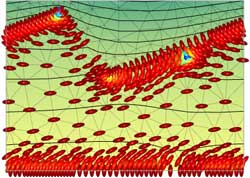Fig. 1: Defect movement in a Zenithally Bistable Nematic (ZBN) style device, with a negative dielectric anisotropy liquid crystal (director colour represents the order parameter)

 T. Qian and P. Sheng, “Generalized hydrodynamic equations for nematic liquid
crystals,” Physical Review E, vol. 58, no. 6, pp. 7475–7485, 1998.

 E. Willman, F. A. Fern´andez, R. James, and S. E. Day, “Phenomenological
anisotropic anchoring energy of nematic liquid crystals for the Landau-de Gennes
theory,” to appear in IEEE Transactions on Electron Devices.

 R. James, E. Willman, F. A. Fernandez, and S. E. Day, “Finite-element modeling
of liquid-crystal hydrodynamics with a variable degree of order,” IEEE Transactions
on Electron Devices, vol. 53, no. 7, pp. 1575–1582, 2006.University College London - Gower Street - London - WC1E 6BT -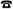+44 (0)20 7679 2000 - Copyright © 1999-2005 UCL